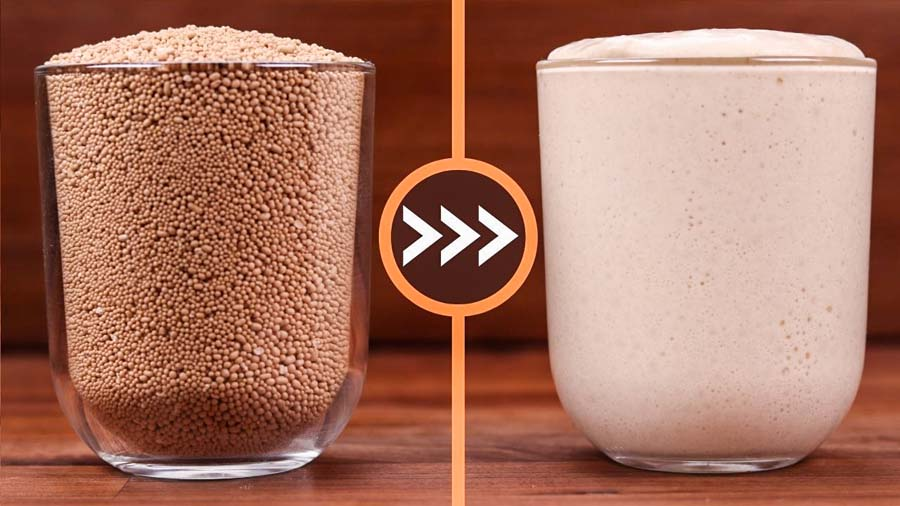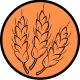# How to Convert Yeast Dough Recipes to be Made With Sourdough

9 9 How to Convert Yeast Dough Recipes to be Made With SourdoughI realized that the ratio of yeast dough to sourdough recipes on my channel is quite unfair, so in this video we will learn how to convert any recipe to a naturally leavened one.

The calculations are similar to my straight dough to yeast preferment conversion. But I have added some extra info here. It is worth watching both videos however because I go into more detail about the types of preferments when it comes to hydration levels.

Also, check out the preferment guide for even more in-depth information.

Baker’s percentage and hydration are two more concepts that you must know in order to be comfortable with converting recipes.

In this video we will concentrate more on the calculations. I take it that if you already have a starter. If not, then check out my starter guide in the Sourdough Bread playlist.

## How I convert a recipe.

Let’s say you have a straight dough recipe with commercial yeast, and you want to convert it. It has 345g white bread flour, 55g whole wheat flour, 8g salt, 260g water, 1g instant yeast.

In baker’s maths that would be: 100% flour, 2% salt, 65% water, 1% yeast. All ingredients are calculated as a percentage in relation to the total amount of flour in the recipe.

The 100% flour is made up of all the flour in the recipe, so the whole 400g.

We will get rid of the dry yeast because we will not be using it.

A standard leaven would be made up of 10% – 20% of the total flour in the recipe. You can choose to ferment any amount of flour that you like. I rarely go less than 10%, but it can easily be way more than 20%. For this example, I chose 15%.

To calculate the amount of flour for the leaven we must find out what 15% of 400g is.

• 400g x 0,15 = 60g

Next, we decide what hydration the leaven should be. I decided to stick to the same hydration as the dough (65%). Baker’s math works the same way with the bulk of the dough as well as the leaven. So, next up we must calculate the amount of water in grams for the leaven.

• 60g x 0,65 = 39g, but we will round this up to 40g just to make things neater, so that the leaven makes up a neat 100g.

Finally, we decide the ratio of the leaven. I know that my starter is active enough to make a leaven rise in 10 hours at a 1:6:4 ratio. That is 1 part sourdough starter, 6 parts flour, 4 parts water.

To calculate each ingredient in grams first add up the leaven ratio values. Then divide the total amount of leaven by that number to find out how much one part of the leaven should weigh.

• 1 + 6 + 4 = 11
• 100 : 11 = 9g (rounded)

Now just multiply the leaven ratio values by 9g.

• 1 x 9 = 9g starter
• 6 x 9 = 54g flour. I will round this to 55g, so that I can use all the wholewheat flour
• 4 x 9 = 36g water
• 9g + 55g + 36g = 100g starter

Note that by now the hydration of the leaven has changed to about 69% because my starter is 100% hydration meaning that it contains 4.5g flour and 4.5g water. But that is not so important. The ratio is the most important part here.

You must however calculate the main dough with these numbers in mind.

Now just subtract the leaven ingredients from the main dough to split the recipe up in its two parts – the preferment and the main dough. This way the hydration of the total formula will still be correct.

## Another way to do it.

Let’s say you have a recipe that is made with a yeast preferment, a poolish.

It contains 100g flour and 100g water, and 0,1g yeast. The hydration makes it a poolish. It has equal parts flour and water making it 100% hydration. For calculation purposes it has only two ingredients, the flour and water. The yeast makes up an insignificant amount.

So, the ratio of this preferment is 1:1.

If we want to turn it into a sourdough poolish we must add the ingredients and then divide by 3 because a leaven will be made up of equal parts starter, flour, and water. 1:1:1

• 200g : 3 = 66g (or thereabout); 66g starter; 66g flour; 66g water.

## How to change the ratio of the leaven.

A poolish is simple because it has the same amounts of ingredients, but other preferments have different ratios and hydration levels.

Let’s say we want to turn this poolish from 1:1:1 to 1:6:4.

All we must do is add up those numbers and then divide the total amount of poolish by that number to find out how much one part of it weighs.

• 1 + 6 + 4 = 11 ; 200g : 11 = 18.18g, but we will round it down to 18g.

One part of this ratio weighs 18g. Now we can multiply each ingredient to find out how many grams it should be.

• 1 x 18 = 18g starter
• 6 x 18 = 108g flour
• 4 x 18 = 72g water

This makes for a total of 198g which is close enough.

The ratio can be changed to whatever, but the calculations will always work the same way.

When I do calculations for my recipes it seems very simple to me, but when I must type them out here is becomes quite complex. I hope all this made sense.

## Perhaps the easiest way to do it.

My method of calculating relies on deciding the percentage of flour in the preferment first. This adds a couple of steps to the whole formula.

Other people like to decide on a percentage of preferment. So, for the previous example they would say that it is 25% preferment.

• 400 x 0.25 = 100

This gets us to the 100g total right away. From here we use the same calculations with the ratios to get our individual ingredients. It makes life simpler, but I just have got used to thinking of it as percentage of total flour in the preferment and it just makes sense to me even though it does add more steps to the calculations.

Watch the video here

Understanding the principles of bread making will let you be in complete control every time you make bread. It will reduce the failure rate and turn you into an even more confident home baker.

I highly recommend you check out the Learning page where I have detailed, easy to understand explanations on each step of the bread baking process and the principles behind it. You can find all the equipment I use and recommend in the Shop (UK) & Shop (US) pages.

L

1.“Let’s say you have a straight dough recipe with commercial yeast, and you want to convert it. It has 345g white bread flour, 55g whole wheat flour, 8g salt, 260g water, 1g instant yeast.

In baker’s maths that would be: 100% flour, 2% salt, 65% water, 1% yeast. All ingredients are calculated as a percentage in relation to the total amount of flour in the recipe.”

Wait! I got lost. I’m still new to this.
I came up with 4g instant yeast is 1% of 400g flour. Or 1g instant yeast is 0.3% of 400g flour. What’d I miss?

•